# NCERT Solutions for Class 9 Science Chapter 9: Force and Laws Of Motion

## NCERT Solutions Class 9 Science Chapter 9 – CBSE Term I Free PDF Download

NCERT Solutions for Class 9 Science Chapter 9 Force and Laws of Motion are prepared with the intention of addressing the students in clearing their doubts and concepts thoroughly. Class 9 Solutions of Science is a beneficial reference material that helps students to clear doubts instantly in an effective way. NCERT Solutions for Class 9 Science approaches students in a student-friendly way and is loaded with questions, activities, and exercises that are CBSE Term I and competitive exam-oriented.  NCERT Solutions for Class 9 Science is designed and developed by the subject experts and teaching faculty having experience in coaching students. It is developed keeping in mind the concept-based approach, along with the precise answering method for examinations. It is a detailed and well-structured concept-based learning solution aimed at imparting confidence to face the CBSE Term I and competitive exam. NCERT Solutions for Class 9 Science is made available in both PDF and web format for ease of access.

## Download PDF of NCERT Solutions for Class 9 Science Chapter 9: Force and Laws Of Motion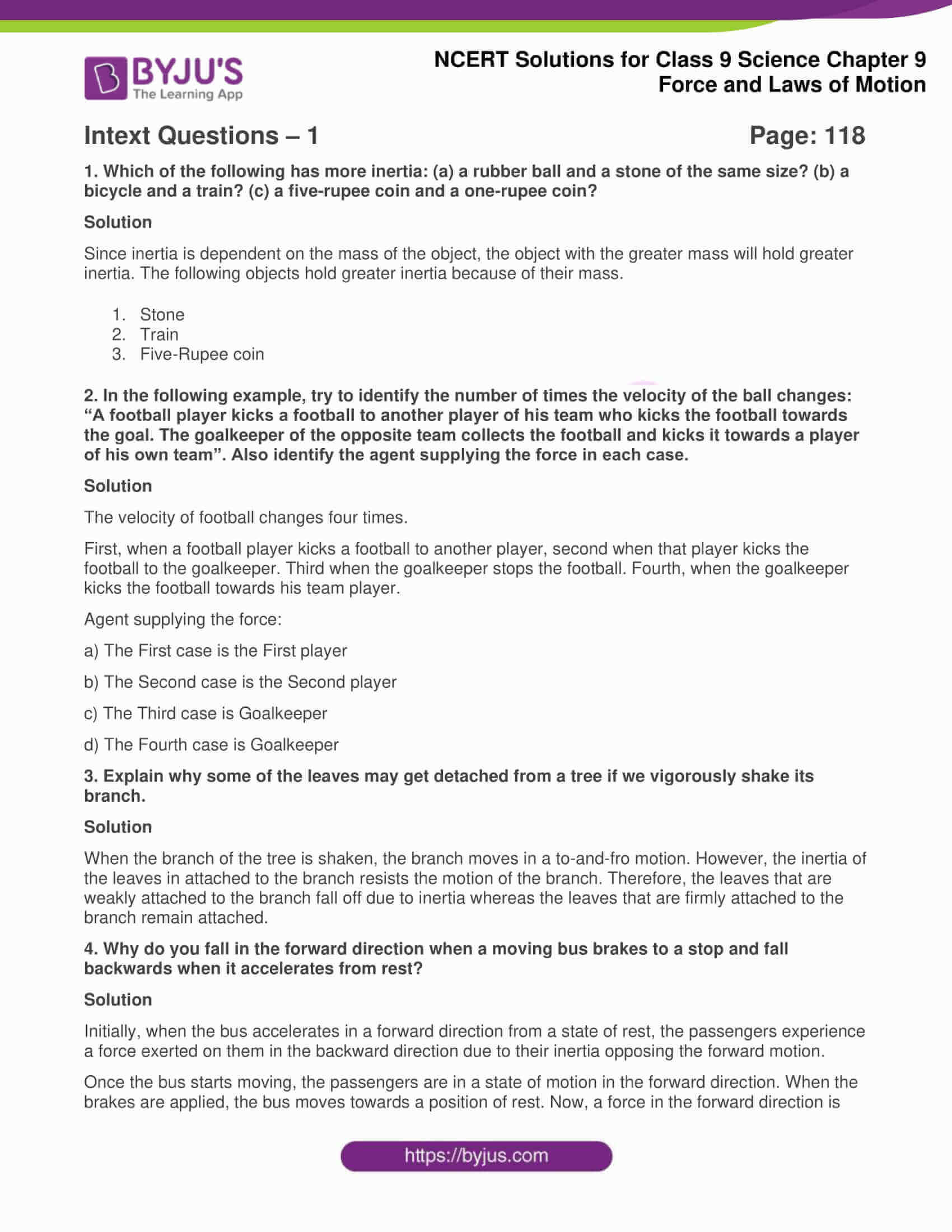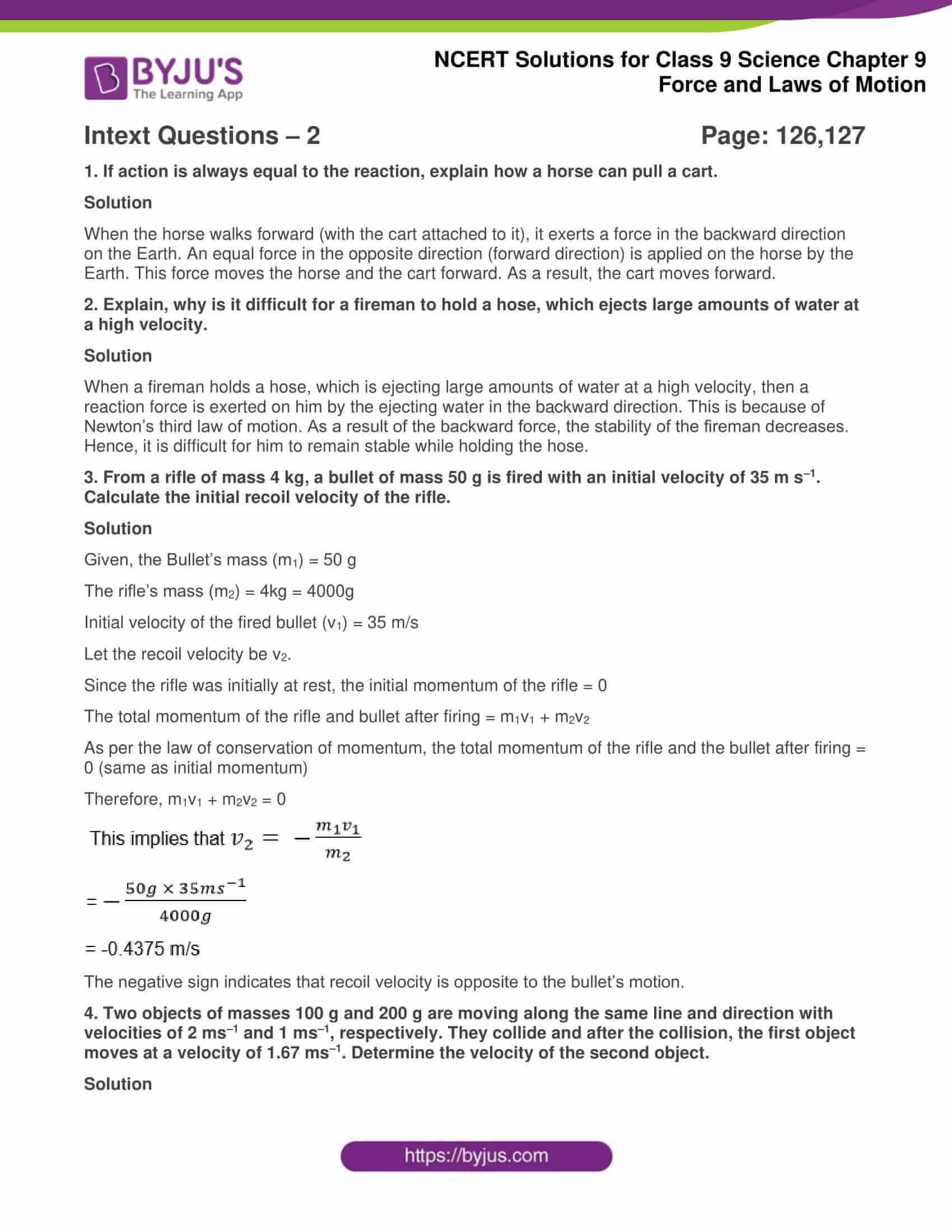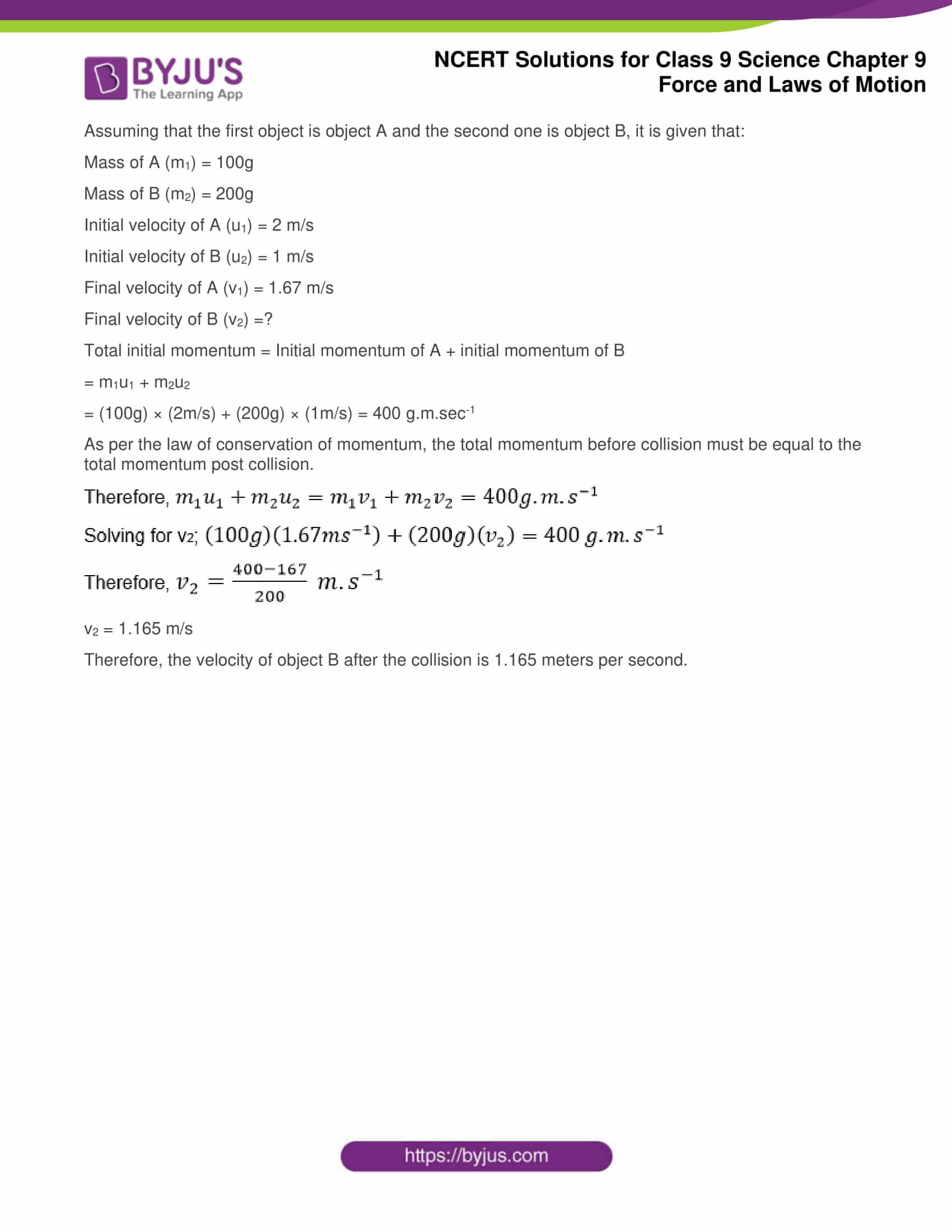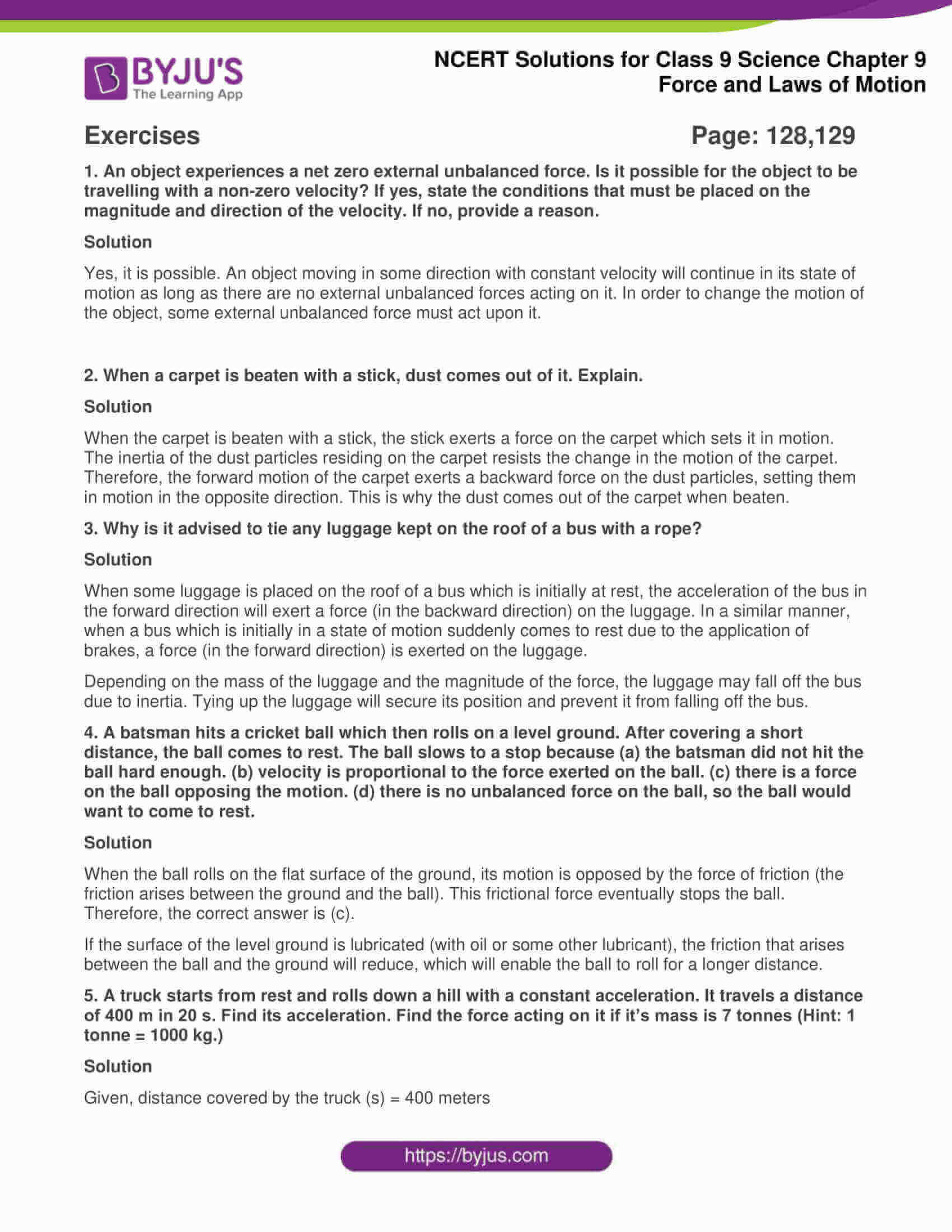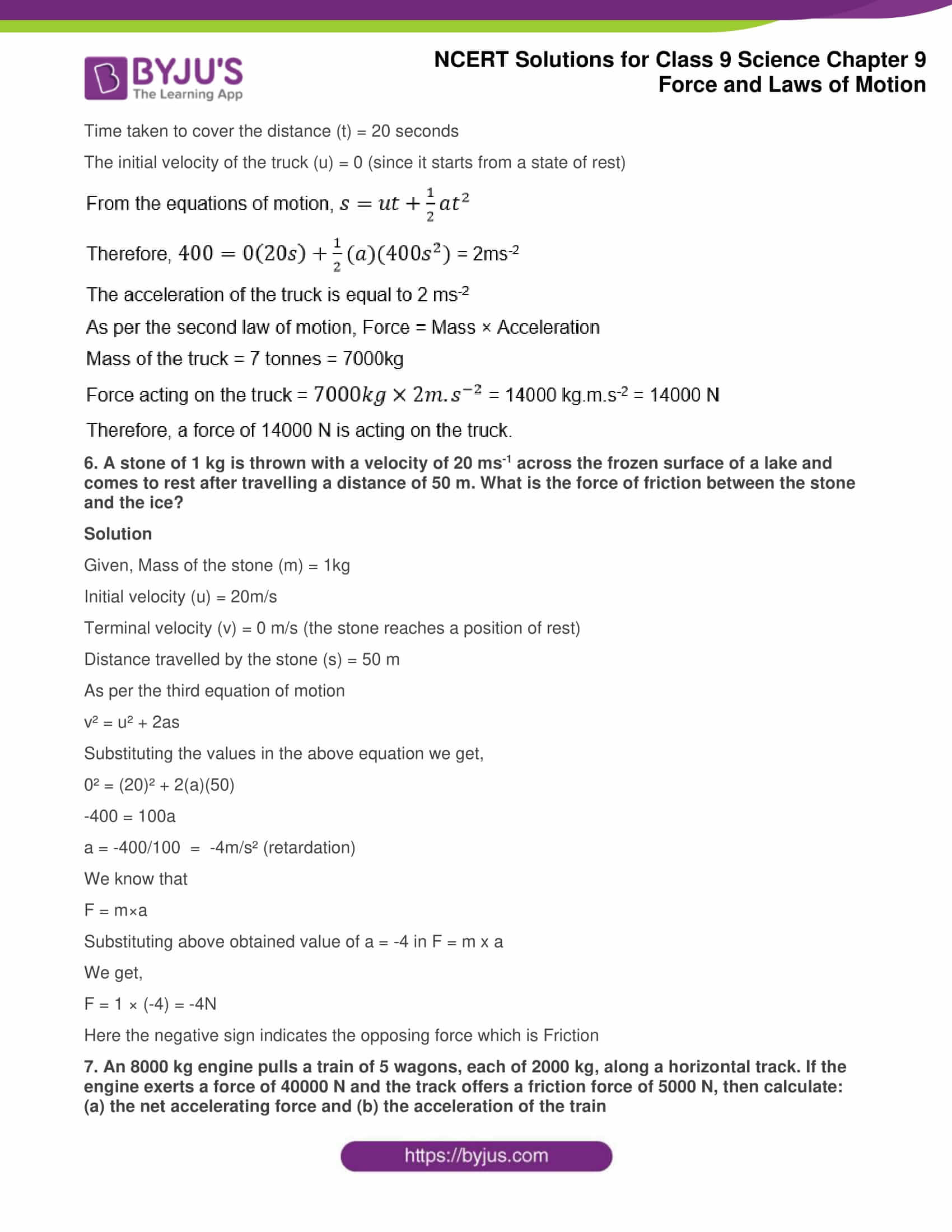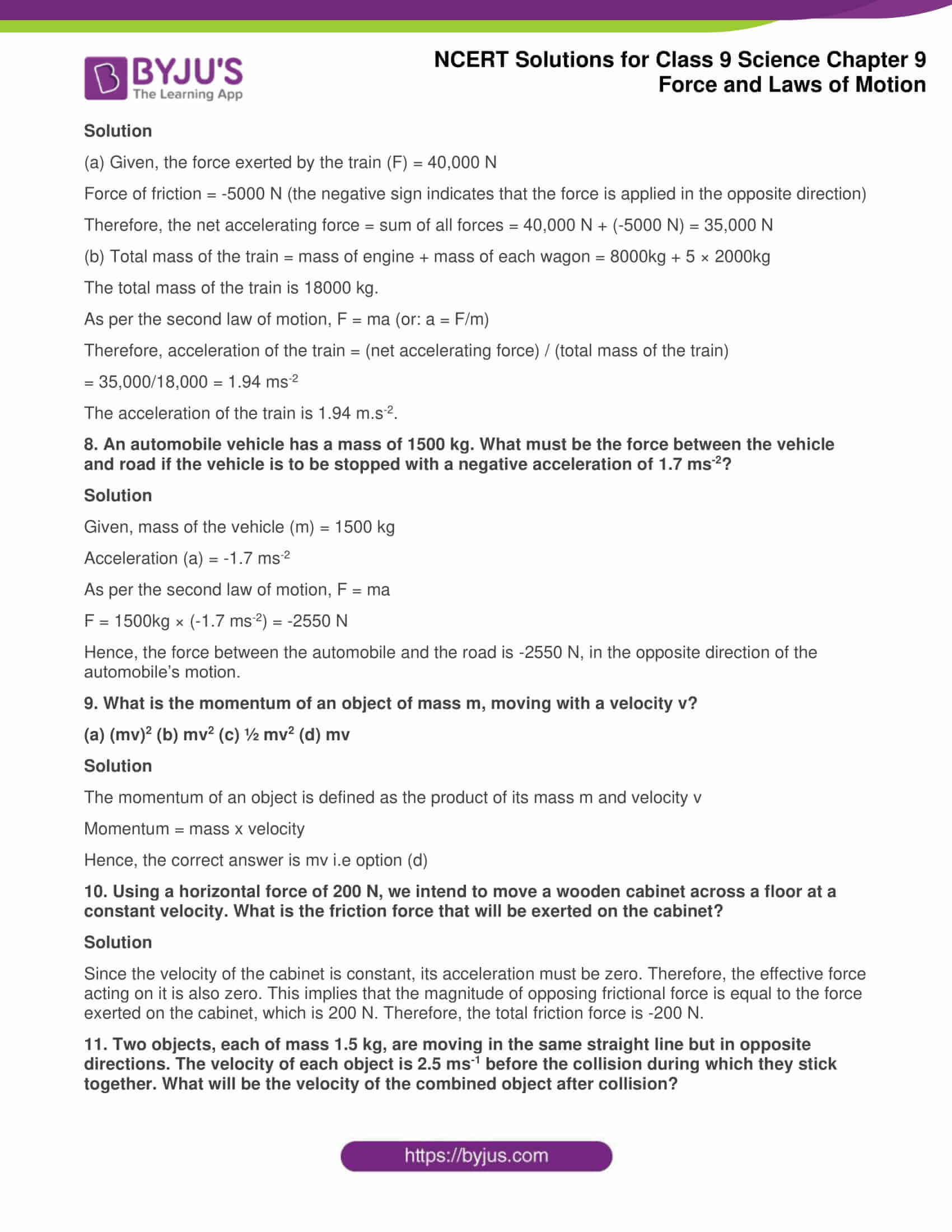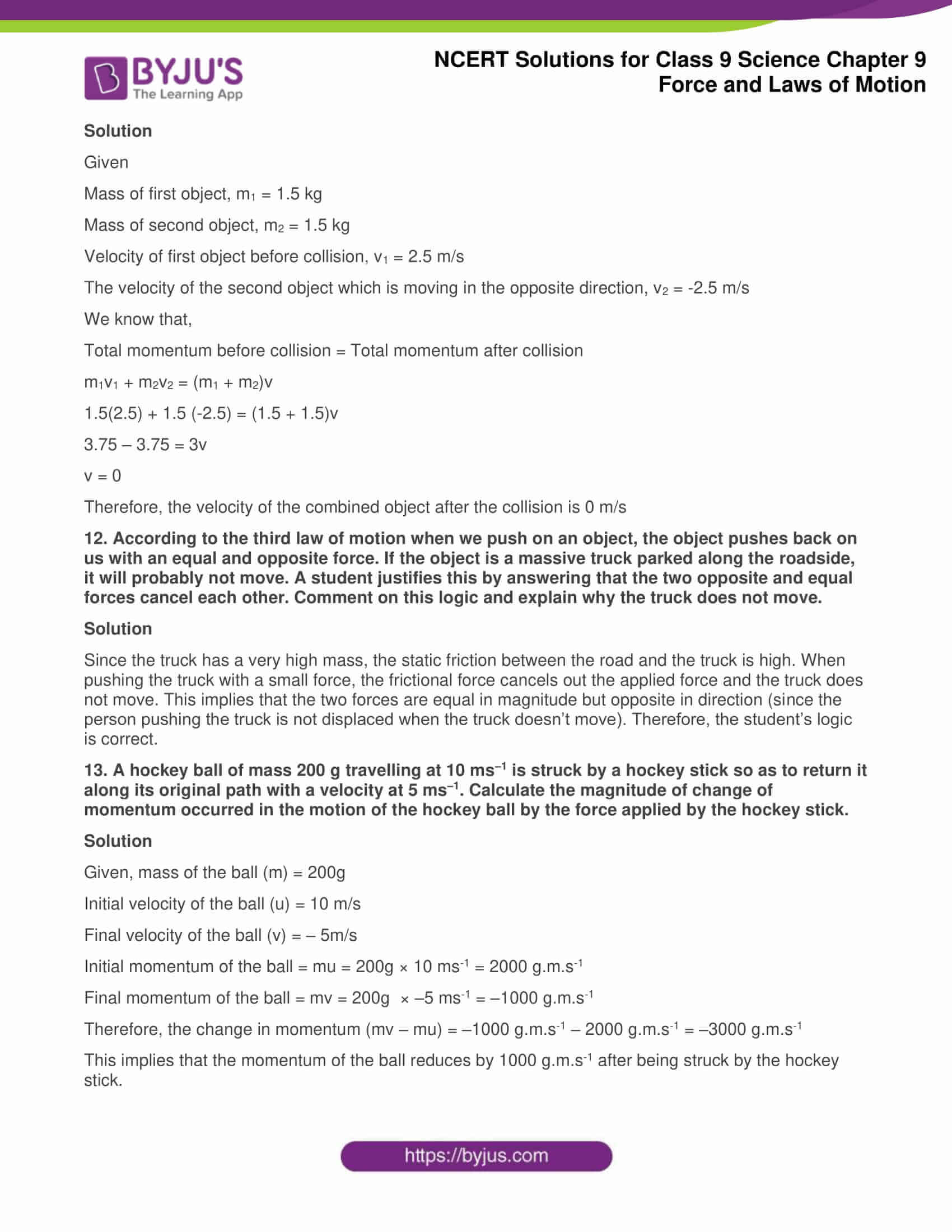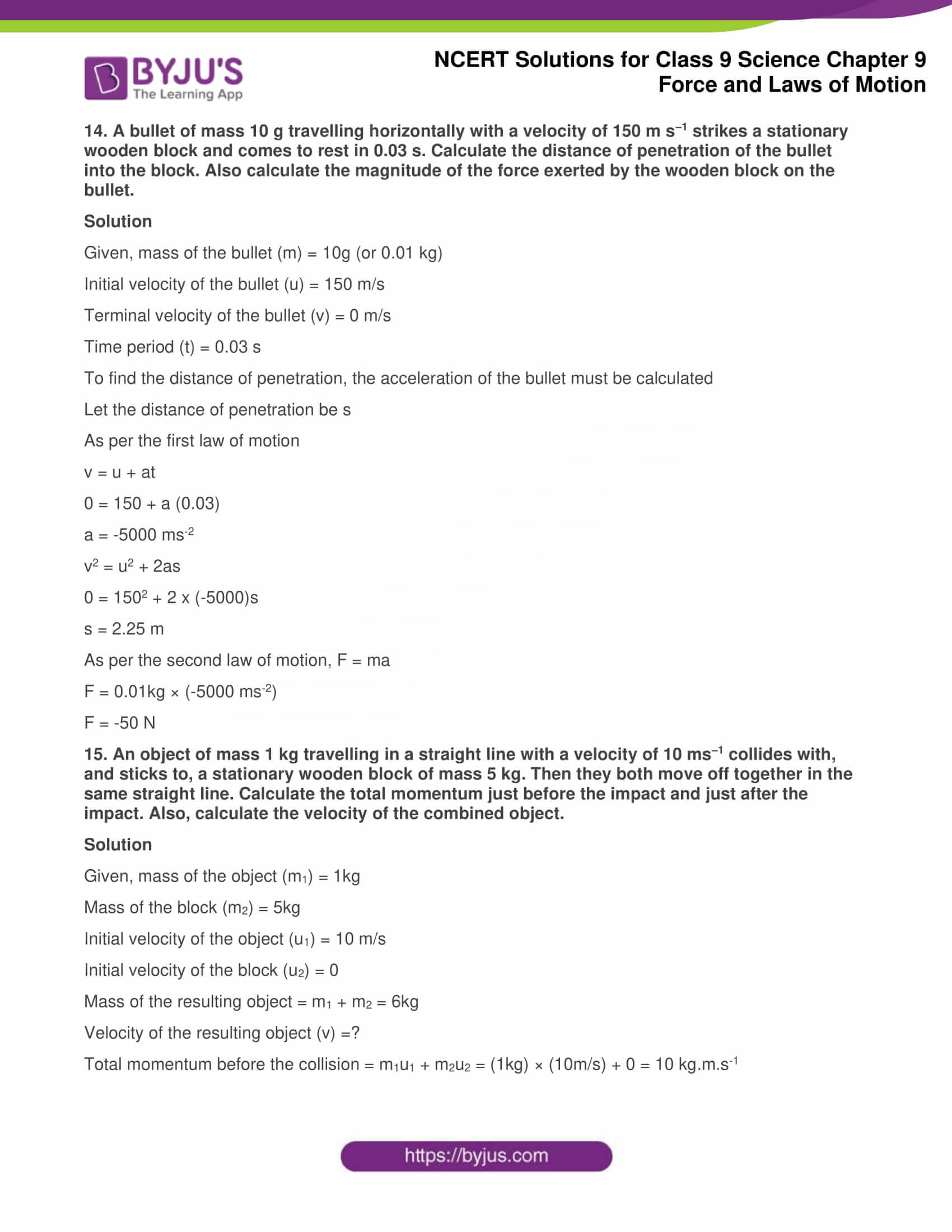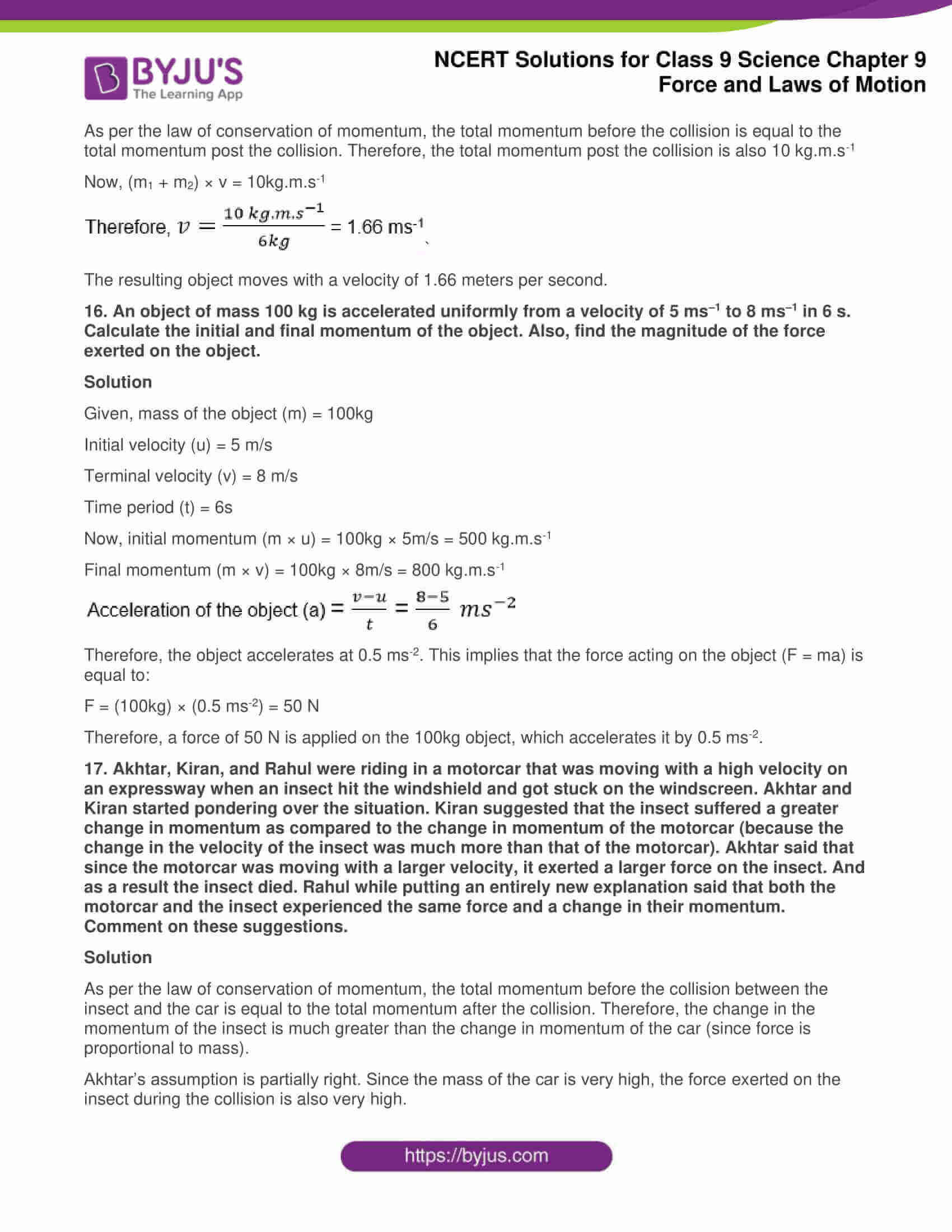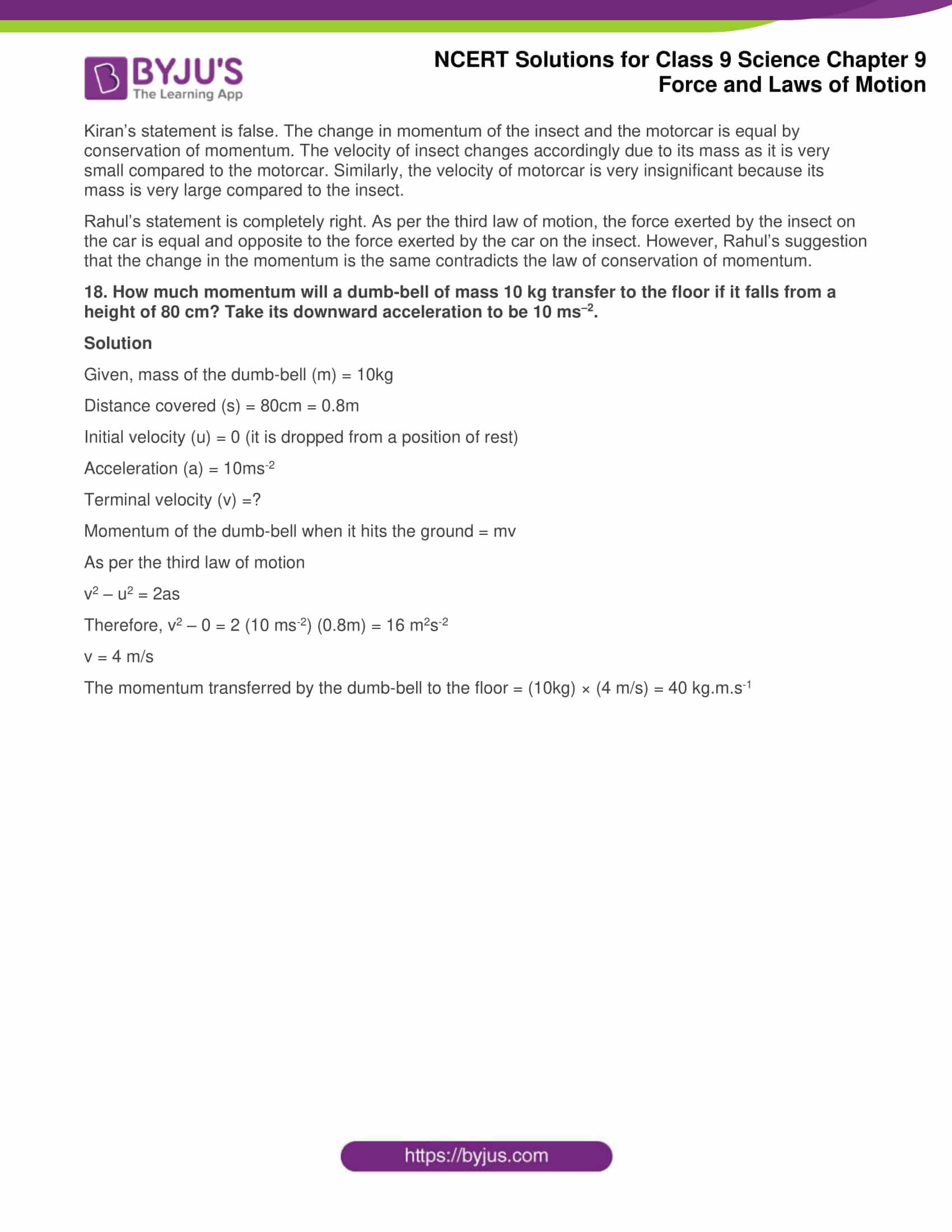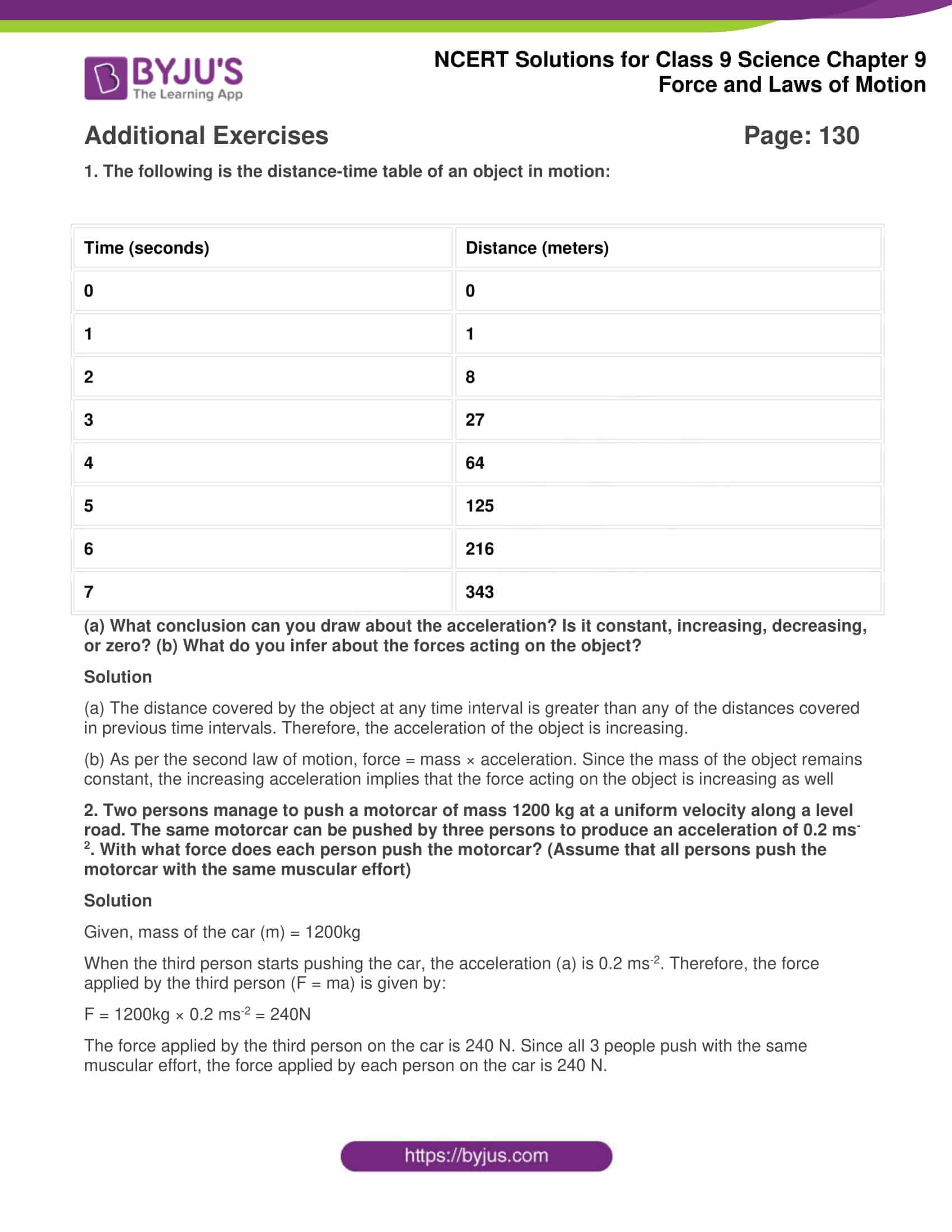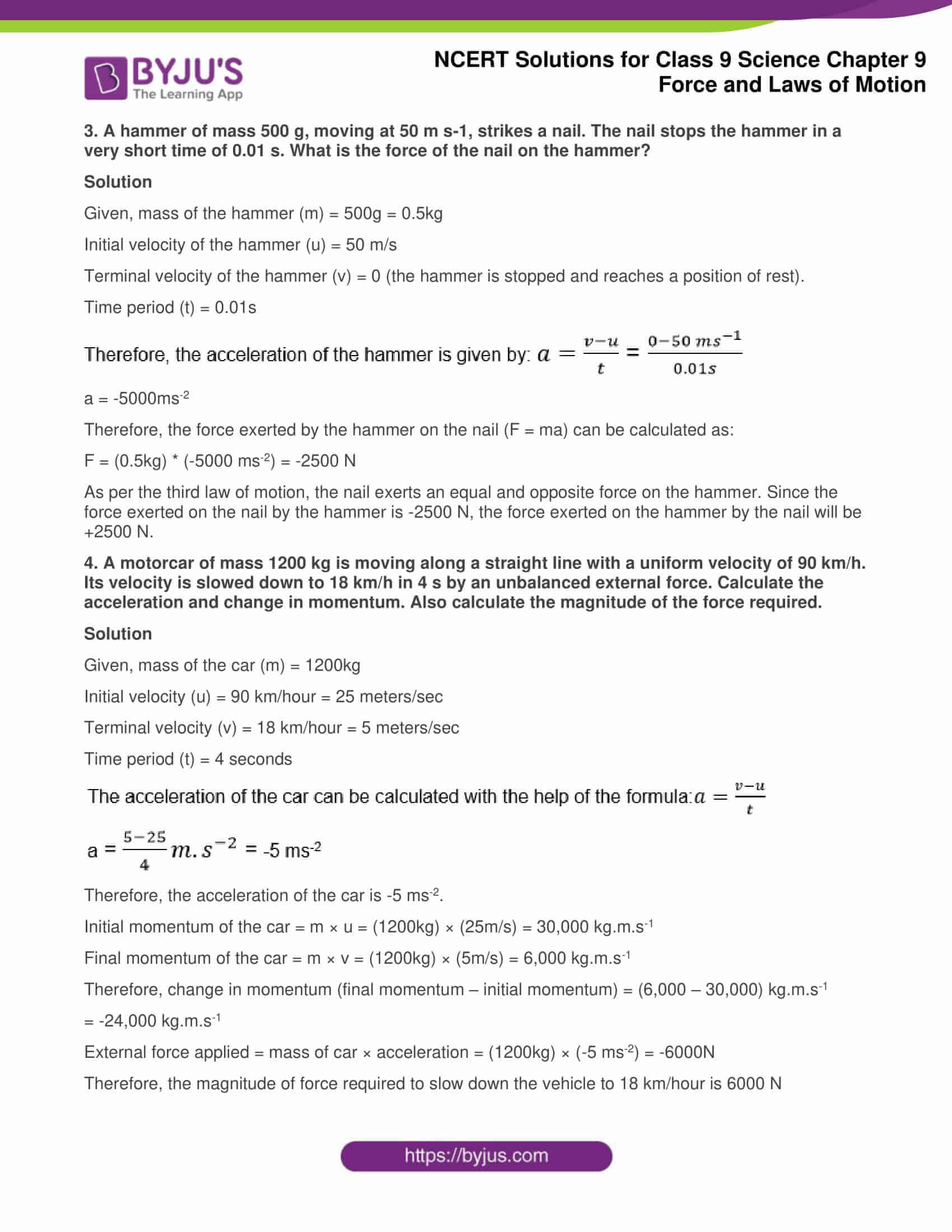### Access Answers of Science NCERT class 9 Chapter 9: Force and Laws Of Motion(All intext and exercise questions solved)

Intext Questions - 1   Page: 118 1. Which of the following has more inertia: (a) a rubber ball and a stone of the same size? (b) a bicycle and a train? (c) a five-rupee coin and a one-rupee coin? Solution Since inertia is dependent on the mass of the object, the object with the greater mass will hold greater inertia. The following objects hold greater inertia because of their mass.
1. Stone
2. Train
3. Five-Rupee coin
2. In the following example, try to identify the number of times the velocity of the ball changes: “A football player kicks a football to another player of his team who kicks the football towards the goal. The goalkeeper of the opposite team collects the football and kicks it towards a player of his own team”. Also identify the agent supplying the force in each case. Solution The velocity of football changes four times. First, when a football player kicks a football to another player, second when that player kicks the football to the goalkeeper. Third when the goalkeeper stops the football. Fourth, when the goalkeeper kicks the football towards his team player. Agent supplying the force: a) The First case is the First player b) The Second case is the Second player c) The Third case is Goalkeeper d) The Fourth case is Goalkeeper 3. Explain why some of the leaves may get detached from a tree if we vigorously shake its branch. Solution When the branch of the tree is shaken, the branch moves in a to-and-fro motion. However, the inertia of the leaves in attached to the branch resists the motion of the branch. Therefore, the leaves that are weakly attached to the branch fall off due to inertia whereas the leaves that are firmly attached to the branch remain attached. 4. Why do you fall in the forward direction when a moving bus brakes to a stop and fall backwards when it accelerates from rest? Solution Initially, when the bus accelerates in a forward direction from a state of rest, the passengers experience a force exerted on them in the backward direction due to their inertia opposing the forward motion. Once the bus starts moving, the passengers are in a state of motion in the forward direction. When the brakes are applied, the bus moves towards a position of rest. Now, a force in the forward direction is applied on the passengers because their inertia resists the change in the motion of the bus. This causes the passengers to fall forwards when the brakes are applied. Intext Questions - 2 Page: 126,127 1. If action is always equal to the reaction, explain how a horse can pull a cart. Solution When the horse walks forward (with the cart attached to it), it exerts a force in the backward direction on the Earth. An equal force in the opposite direction (forward direction) is applied on the horse by the Earth. This force moves the horse and the cart forward. As a result, the cart moves forward. 2. Explain, why is it difficult for a fireman to hold a hose, which ejects large amounts of water at a high velocity. Solution When a fireman holds a hose, which is ejecting large amounts of water at a high velocity, then a reaction force is exerted on him by the ejecting water in the backward direction. This is because of Newton’s third law of motion. As a result of the backward force, the stability of the fireman decreases. Hence, it is difficult for him to remain stable while holding the hose. 3. From a rifle of mass 4 kg, a bullet of mass 50 g is fired with an initial velocity of 35 m s–1. Calculate the initial recoil velocity of the rifle. Solution Given, the Bullet’s mass (m1) = 50 g The rifle’s mass (m2) = 4kg = 4000g Initial velocity of the fired bullet (v1) = 35 m/s Let the recoil velocity be v2. Since the rifle was initially at rest, the initial momentum of the rifle = 0 The total momentum of the rifle and bullet after firing = m1v1 + m2v2 As per the law of conservation of momentum, the total momentum of the rifle and the bullet after firing = 0 (same as initial momentum) Therefore, m1v1 + m2v2 = 0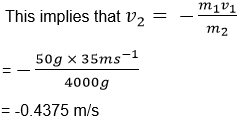The negative sign indicates that recoil velocity is opposite to the bullet's motion. 4. Two objects of masses 100 g and 200 g are moving along the same line and direction with velocities of 2 ms–1 and 1 ms–1, respectively. They collide and after the collision, the first object moves at a velocity of 1.67 ms–1. Determine the velocity of the second object. Solution Assuming that the first object is object A and the second one is object B, it is given that: Mass of A (m1) = 100g Mass of B (m2) = 200g Initial velocity of A (u1) = 2 m/s Initial velocity of B (u2) = 1 m/s Final velocity of A (v1) = 1.67 m/s Final velocity of B (v2) =? Total initial momentum = Initial momentum of A + initial momentum of B = m1u1 + m2u2 = (100g) × (2m/s) + (200g) × (1m/s) = 400 g.m.sec-1 As per the law of conservation of momentum, the total momentum before collision must be equal to the total momentum post collision.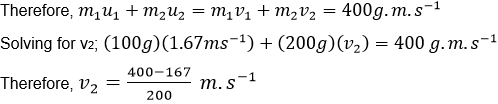v2 = 1.165 m/s Therefore, the velocity of object B after the collision is 1.165 meters per second. Exercises Page: 128,129 1. An object experiences a net zero external unbalanced force. Is it possible for the object to be travelling with a non-zero velocity? If yes, state the conditions that must be placed on the magnitude and direction of the velocity. If no, provide a reason. Solution Yes, it is possible. An object moving in some direction with constant velocity will continue in its state of motion as long as there are no external unbalanced forces acting on it. In order to change the motion of the object, some external unbalanced force must act upon it.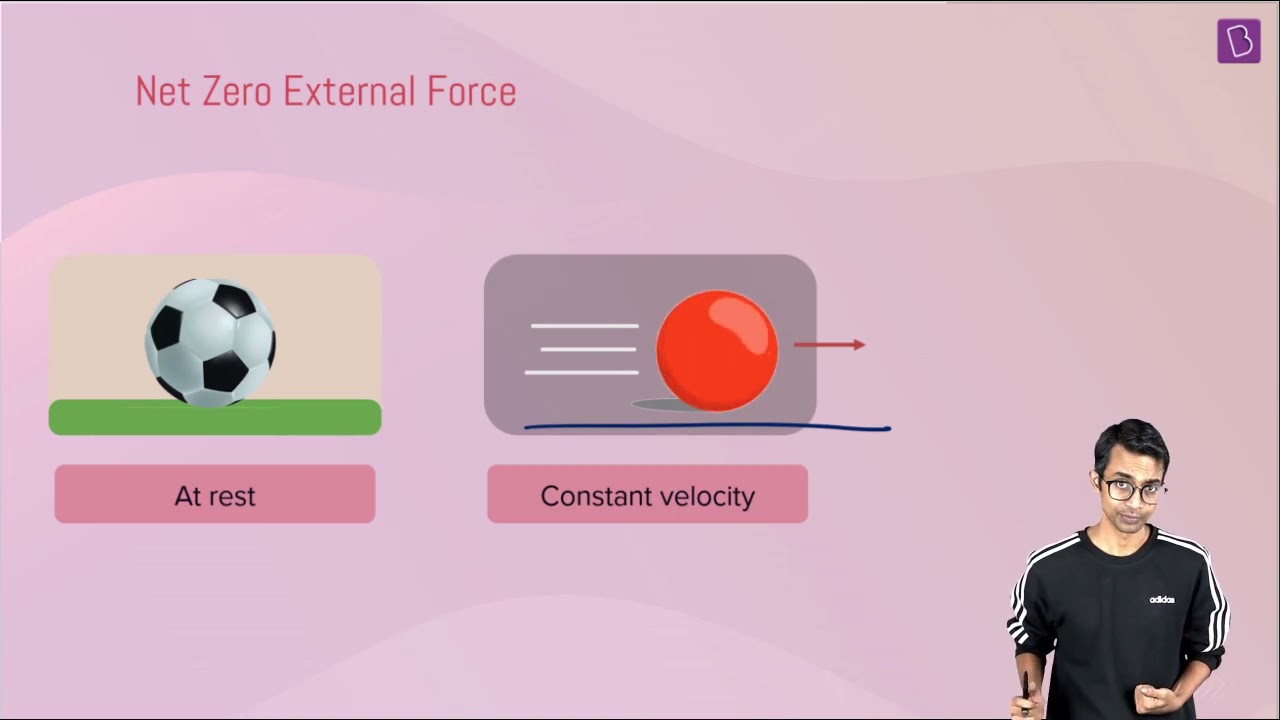2. When a carpet is beaten with a stick, dust comes out of it. Explain. Solution When the carpet is beaten with a stick, the stick exerts a force on the carpet which sets it in motion. The inertia of the dust particles residing on the carpet resists the change in the motion of the carpet. Therefore, the forward motion of the carpet exerts a backward force on the dust particles, setting them in motion in the opposite direction. This is why the dust comes out of the carpet when beaten. 3. Why is it advised to tie any luggage kept on the roof of a bus with a rope? Solution When some luggage is placed on the roof of a bus which is initially at rest, the acceleration of the bus in the forward direction will exert a force (in the backward direction) on the luggage. In a similar manner, when a bus which is initially in a state of motion suddenly comes to rest due to the application of brakes, a force (in the forward direction) is exerted on the luggage. Depending on the mass of the luggage and the magnitude of the force, the luggage may fall off the bus due to inertia. Tying up the luggage will secure its position and prevent it from falling off the bus.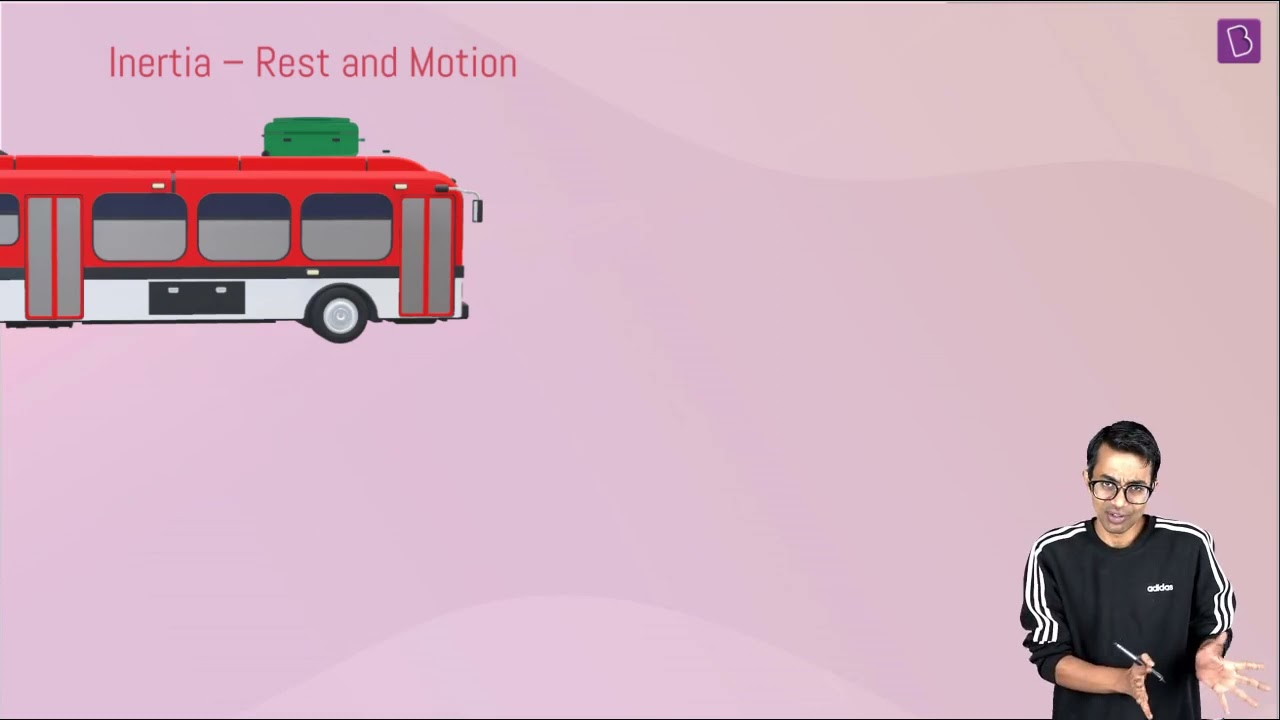4. A batsman hits a cricket ball which then rolls on a level ground. After covering a short distance, the ball comes to rest. The ball slows to a stop because (a) the batsman did not hit the ball hard enough. (b) velocity is proportional to the force exerted on the ball. (c) there is a force on the ball opposing the motion. (d) there is no unbalanced force on the ball, so the ball would want to come to rest. Solution When the ball rolls on the flat surface of the ground, its motion is opposed by the force of friction (the friction arises between the ground and the ball). This frictional force eventually stops the ball. Therefore, the correct answer is (c). If the surface of the level ground is lubricated (with oil or some other lubricant), the friction that arises between the ball and the ground will reduce, which will enable the ball to roll for a longer distance. 5. A truck starts from rest and rolls down a hill with a constant acceleration. It travels a distance of 400 m in 20 s. Find its acceleration. Find the force acting on it if it’s mass is 7 tonnes (Hint: 1 tonne = 1000 kg.) Solution Given, distance covered by the truck (s) = 400 meters Time taken to cover the distance (t) = 20 seconds The initial velocity of the truck (u) = 0 (since it starts from a state of rest)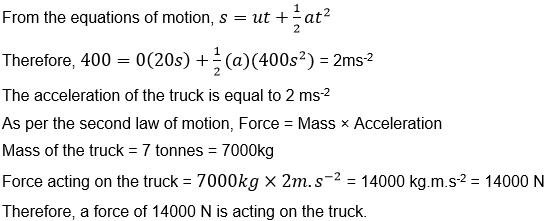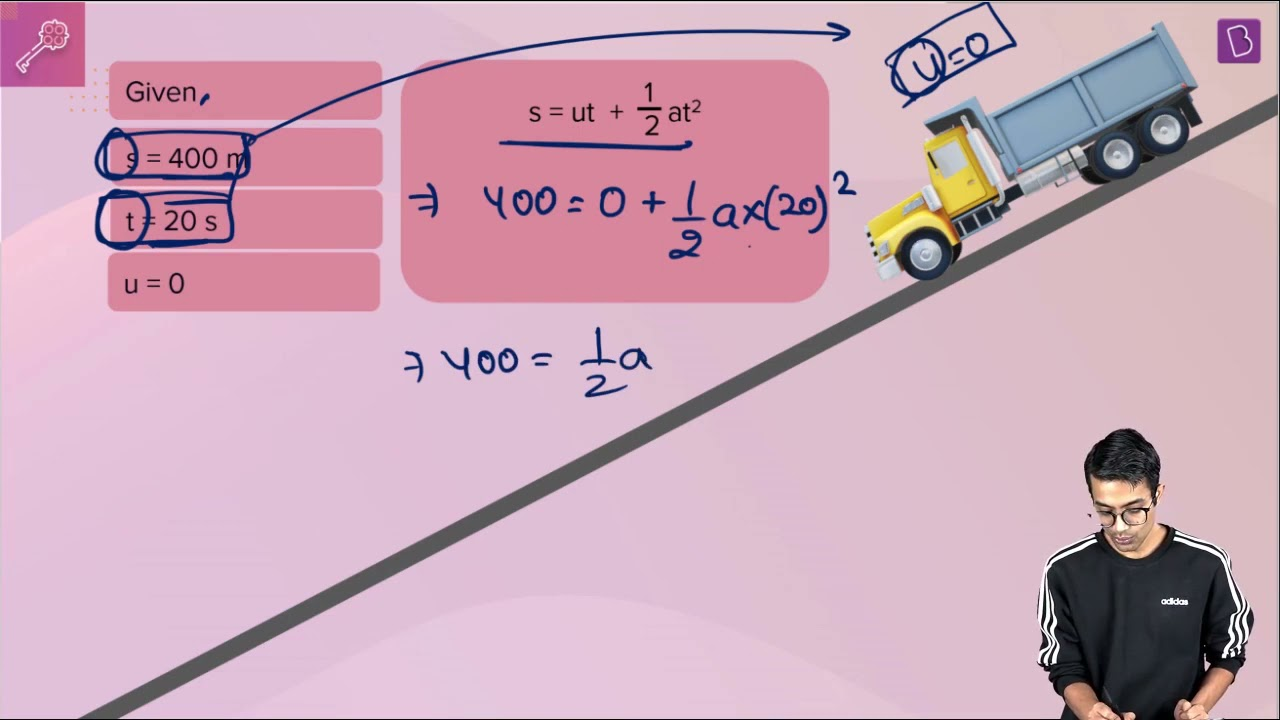6. A stone of 1 kg is thrown with a velocity of 20 ms-1 across the frozen surface of a lake and comes to rest after travelling a distance of 50 m. What is the force of friction between the stone and the ice? Solution Given, Mass of the stone (m) = 1kg Initial velocity (u) = 20m/s Terminal velocity (v) = 0 m/s (the stone reaches a position of rest) Distance travelled by the stone (s) = 50 m As per the third equation of motion v² = u² + 2as Substituting the values in the above equation we get, 0² = (20)² + 2(a)(50) -400 = 100a a = -400/100  =  -4m/s² (retardation) We know that F = m×a Substituting above obtained value of a = -4 in F = m x a We get, F = 1 × (-4) = -4N Here the negative sign indicates the opposing force which is Friction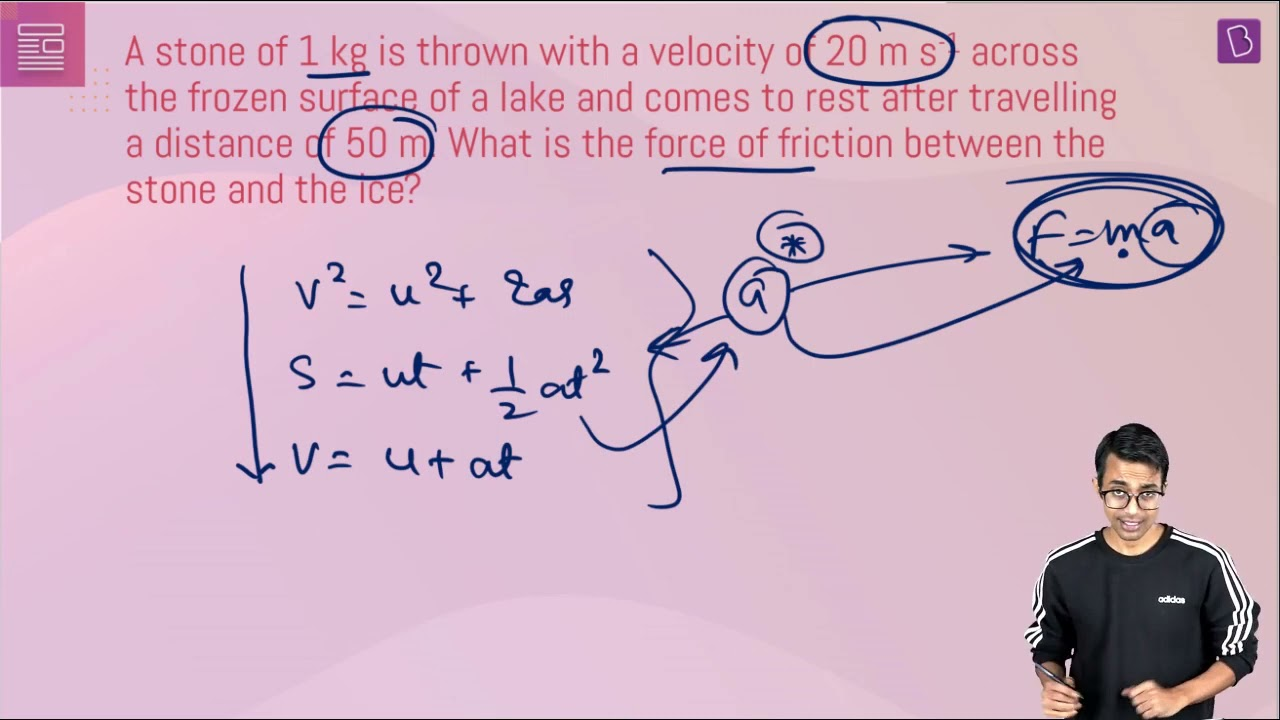7. An 8000 kg engine pulls a train of 5 wagons, each of 2000 kg, along a horizontal track. If the engine exerts a force of 40000 N and the track offers a friction force of 5000 N, then calculate: (a) the net accelerating force and (b) the acceleration of the train Solution (a) Given, the force exerted by the train (F) = 40,000 N Force of friction = -5000 N (the negative sign indicates that the force is applied in the opposite direction) Therefore, the net accelerating force = sum of all forces = 40,000 N + (-5000 N) = 35,000 N (b) Total mass of the train = mass of engine + mass of each wagon = 8000kg + 5 × 2000kg The total mass of the train is 18000 kg. As per the second law of motion, F = ma (or: a = F/m) Therefore, acceleration of the train = (net accelerating force) / (total mass of the train) = 35,000/18,000 = 1.94 ms-2 The acceleration of the train is 1.94 m.s-2.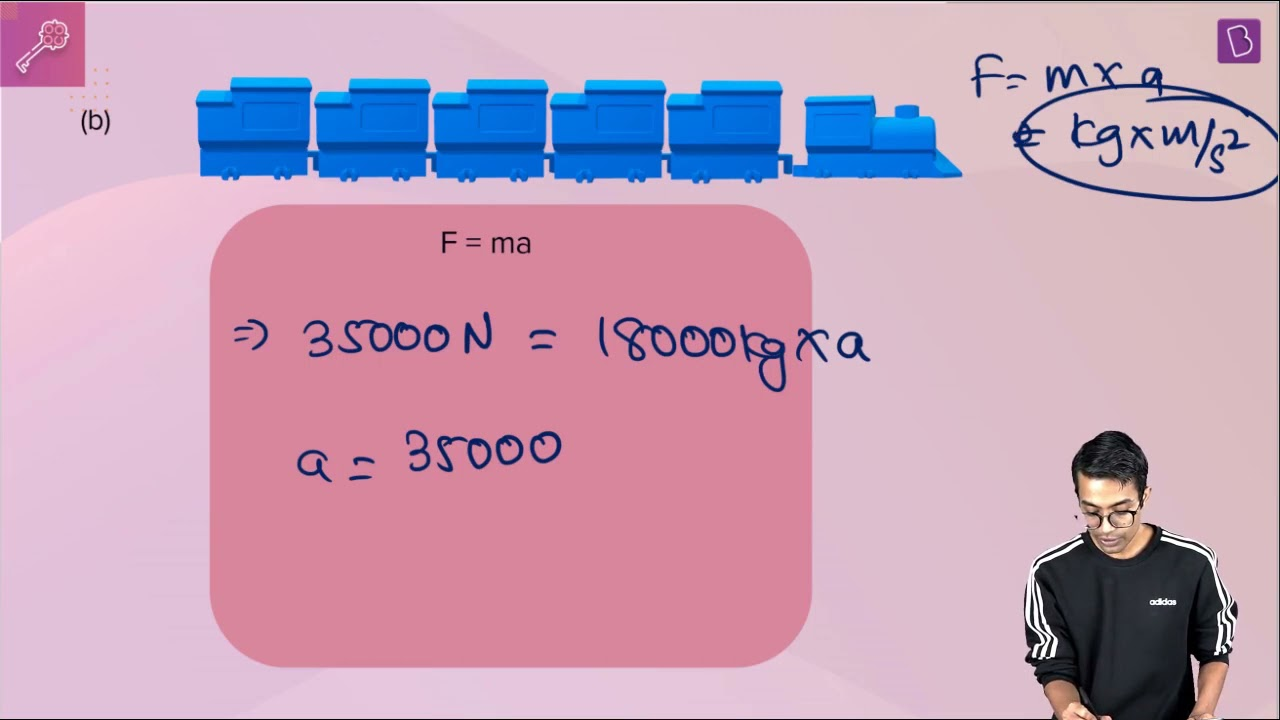8. An automobile vehicle has a mass of 1500 kg. What must be the force between the vehicle and road if the vehicle is to be stopped with a negative acceleration of 1.7 ms-2? Solution Given, mass of the vehicle (m) = 1500 kg Acceleration (a) = -1.7 ms-2 As per the second law of motion, F = ma F = 1500kg × (-1.7 ms-2) = -2550 N Hence, the force between the automobile and the road is -2550 N, in the opposite direction of the automobile’s motion.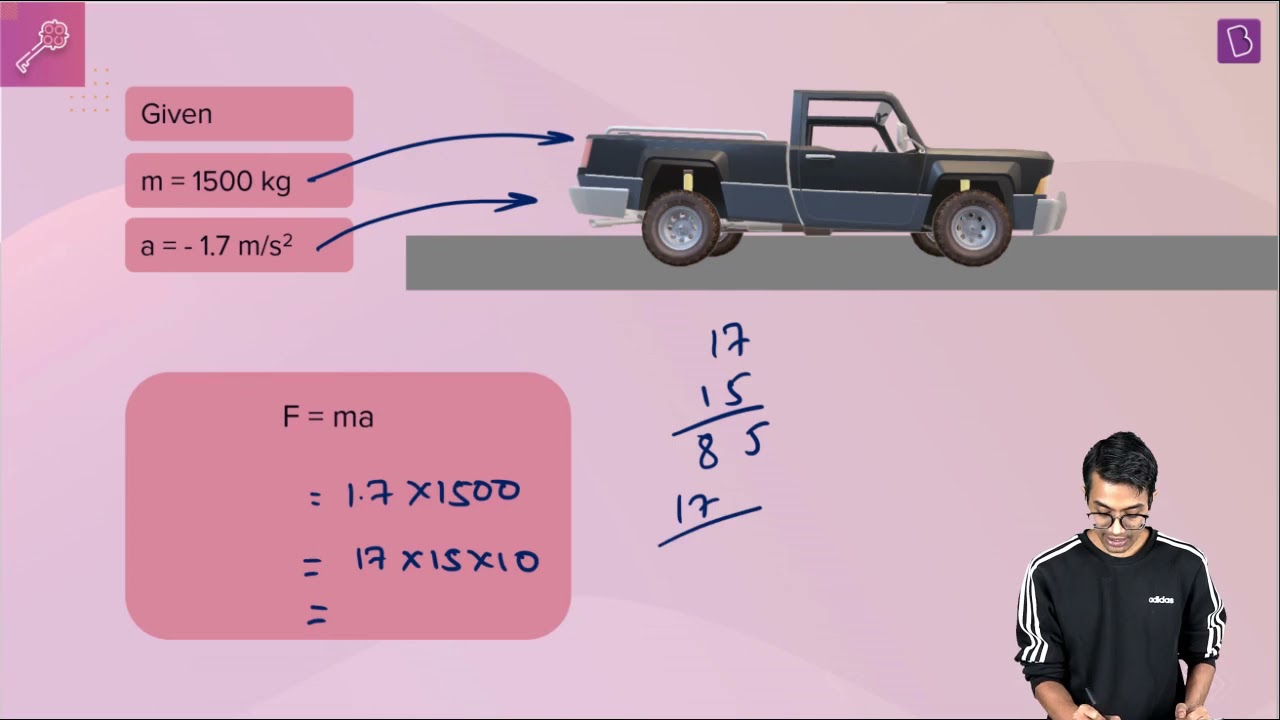9. What is the momentum of an object of mass m, moving with a velocity v? (a) (mv)2 (b) mv2 (c) ½ mv2 (d) mv Solution The momentum of an object is defined as the product of its mass m and velocity v Momentum = mass x velocity Hence, the correct answer is mv i.e option (d) 10. Using a horizontal force of 200 N, we intend to move a wooden cabinet across a floor at a constant velocity. What is the friction force that will be exerted on the cabinet? Solution Since the velocity of the cabinet is constant, its acceleration must be zero. Therefore, the effective force acting on it is also zero. This implies that the magnitude of opposing frictional force is equal to the force exerted on the cabinet, which is 200 N. Therefore, the total friction force is -200 N.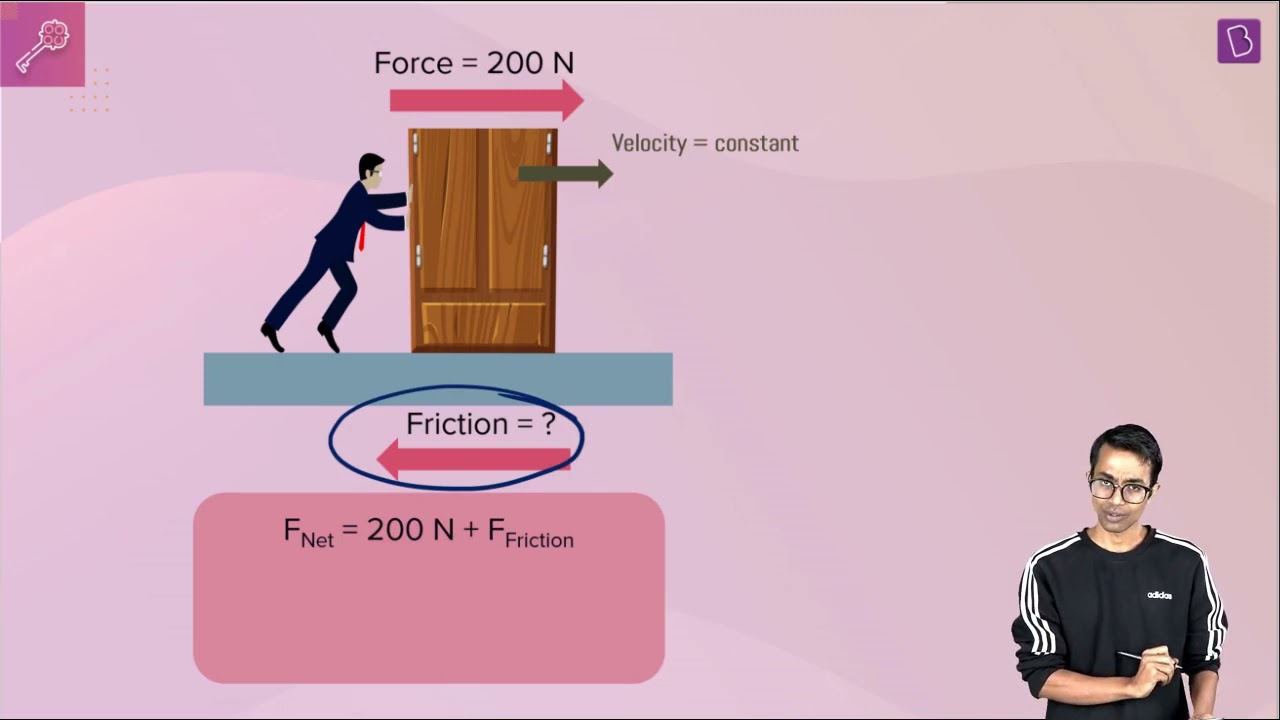11. Two objects, each of mass 1.5 kg, are moving in the same straight line but in opposite directions. The velocity of each object is 2.5 ms-1 before the collision during which they stick together. What will be the velocity of the combined object after collision? Solution Given Mass of first object, m1 = 1.5 kg Mass of second object, m2 = 1.5 kg Velocity of first object before collision, v1 = 2.5 m/s The velocity of the second object which is moving in the opposite direction, v2 = -2.5 m/s We know that, Total momentum before collision = Total momentum after collision m1v1 + m2v2 = (m1 + m2)v 1.5(2.5) + 1.5 (-2.5) = (1.5 + 1.5)v 3.75 - 3.75 = 3v v = 0 Therefore, the velocity of the combined object after the collision is 0 m/s 12. According to the third law of motion when we push on an object, the object pushes back on us with an equal and opposite force. If the object is a massive truck parked along the roadside, it will probably not move. A student justifies this by answering that the two opposite and equal forces cancel each other. Comment on this logic and explain why the truck does not move. Solution Since the truck has a very high mass, the static friction between the road and the truck is high. When pushing the truck with a small force, the frictional force cancels out the applied force and the truck does not move. This implies that the two forces are equal in magnitude but opposite in direction (since the person pushing the truck is not displaced when the truck doesn’t move). Therefore, the student’s logic is correct.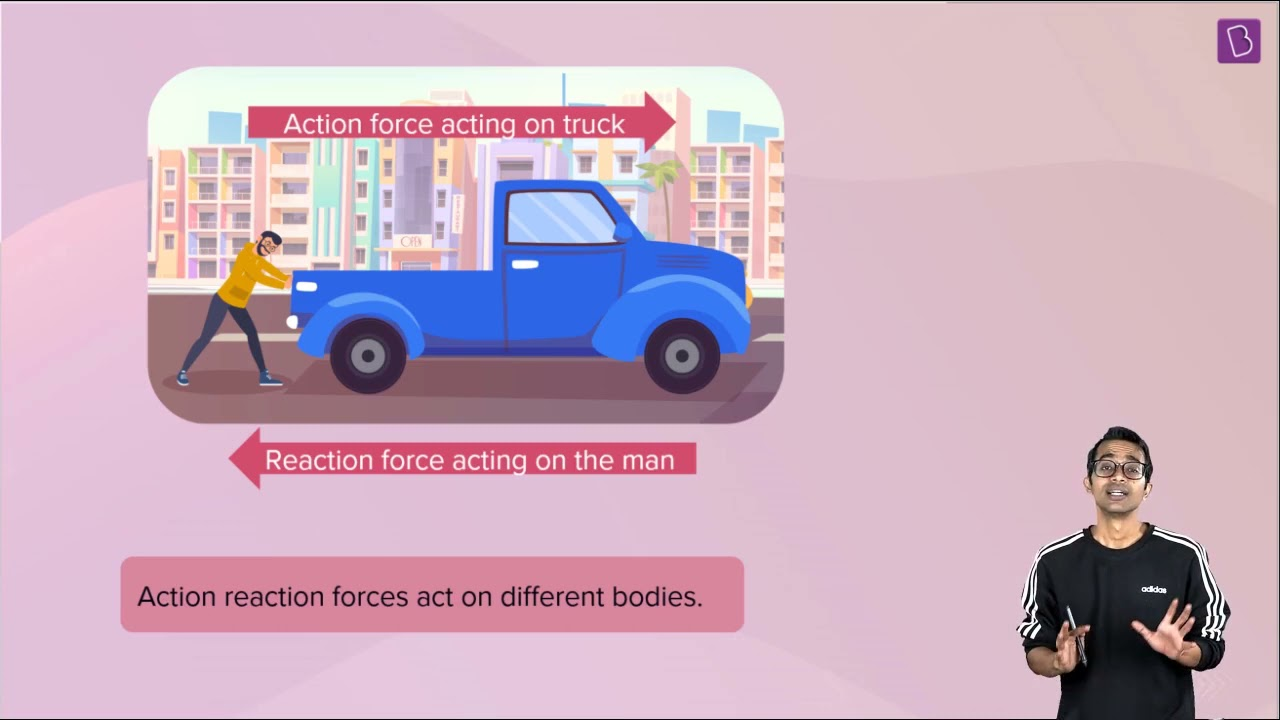13. A hockey ball of mass 200 g travelling at 10 ms–1 is struck by a hockey stick so as to return it along its original path with a velocity at 5 ms–1. Calculate the magnitude of change of momentum occurred in the motion of the hockey ball by the force applied by the hockey stick. Solution Given, mass of the ball (m) = 200g Initial velocity of the ball (u) = 10 m/s Final velocity of the ball (v) = – 5m/s Initial momentum of the ball = mu = 200g × 10 ms-1 = 2000 g.m.s-1 Final momentum of the ball = mv = 200g  × –5 ms-1 = –1000 g.m.s-1 Therefore, the change in momentum (mv – mu) = –1000 g.m.s-1 - 2000 g.m.s-1 = –3000 g.m.s-1 This implies that the momentum of the ball reduces by 1000 g.m.s-1 after being struck by the hockey stick. 14. A bullet of mass 10 g travelling horizontally with a velocity of 150 m s–1 strikes a stationary wooden block and comes to rest in 0.03 s. Calculate the distance of penetration of the bullet into the block. Also calculate the magnitude of the force exerted by the wooden block on the bullet. Solution Given, mass of the bullet (m) = 10g (or 0.01 kg) Initial velocity of the bullet (u) = 150 m/s Terminal velocity of the bullet (v) = 0 m/s Time period (t) = 0.03 s To find the distance of penetration, the acceleration of the bullet must be calculated Let the distance of penetration be s As per the first law of motion v = u + at 0 = 150 + a (0.03) a = -5000 ms-2 v2 = u2 + 2as 0 = 1502 + 2 x (-5000)s s = 2.25 m As per the second law of motion, F = ma F = 0.01kg × (-5000 ms-2) F = -50 N 15. An object of mass 1 kg travelling in a straight line with a velocity of 10 ms–1 collides with, and sticks to, a stationary wooden block of mass 5 kg. Then they both move off together in the same straight line. Calculate the total momentum just before the impact and just after the impact. Also, calculate the velocity of the combined object. Solution Given, mass of the object (m1) = 1kg Mass of the block (m2) = 5kg Initial velocity of the object (u1) = 10 m/s Initial velocity of the block (u2) = 0 Mass of the resulting object = m1 + m2 = 6kg Velocity of the resulting object (v) =? Total momentum before the collision = m1u1 + m2u2 = (1kg) × (10m/s) + 0 = 10 kg.m.s-1 As per the law of conservation of momentum, the total momentum before the collision is equal to the total momentum post the collision. Therefore, the total momentum post the collision is also 10 kg.m.s-1 Now, (m1 + m2) × v = 10kg.m.s-1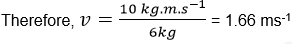` The resulting object moves with a velocity of 1.66 meters per second.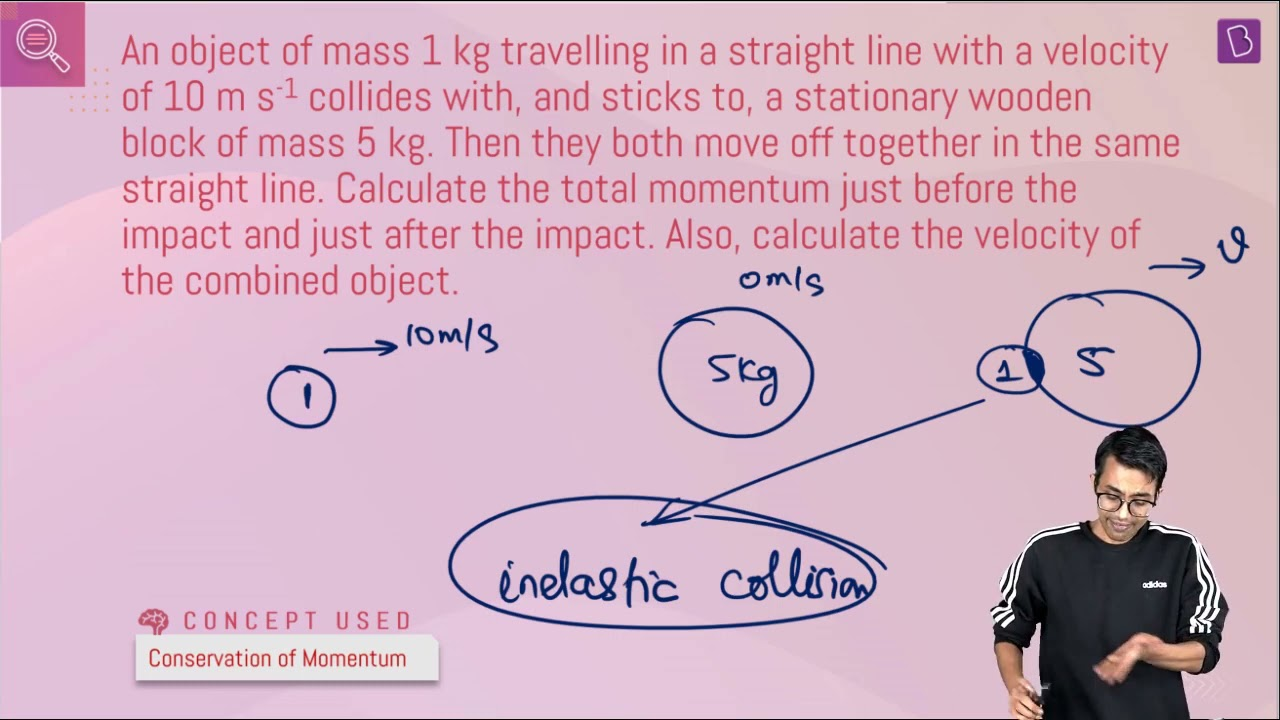16. An object of mass 100 kg is accelerated uniformly from a velocity of 5 ms–1 to 8 ms–1 in 6 s. Calculate the initial and final momentum of the object. Also, find the magnitude of the force exerted on the object. Solution Given, mass of the object (m) = 100kg Initial velocity (u) = 5 m/s Terminal velocity (v) = 8 m/s Time period (t) = 6s Now, initial momentum (m × u) = 100kg × 5m/s = 500 kg.m.s-1 Final momentum (m × v) = 100kg × 8m/s = 800 kg.m.s-1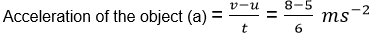Therefore, the object accelerates at 0.5 ms-2. This implies that the force acting on the object (F = ma) is equal to: F = (100kg) × (0.5 ms-2) = 50 N Therefore, a force of 50 N is applied on the 100kg object, which accelerates it by 0.5 ms-2.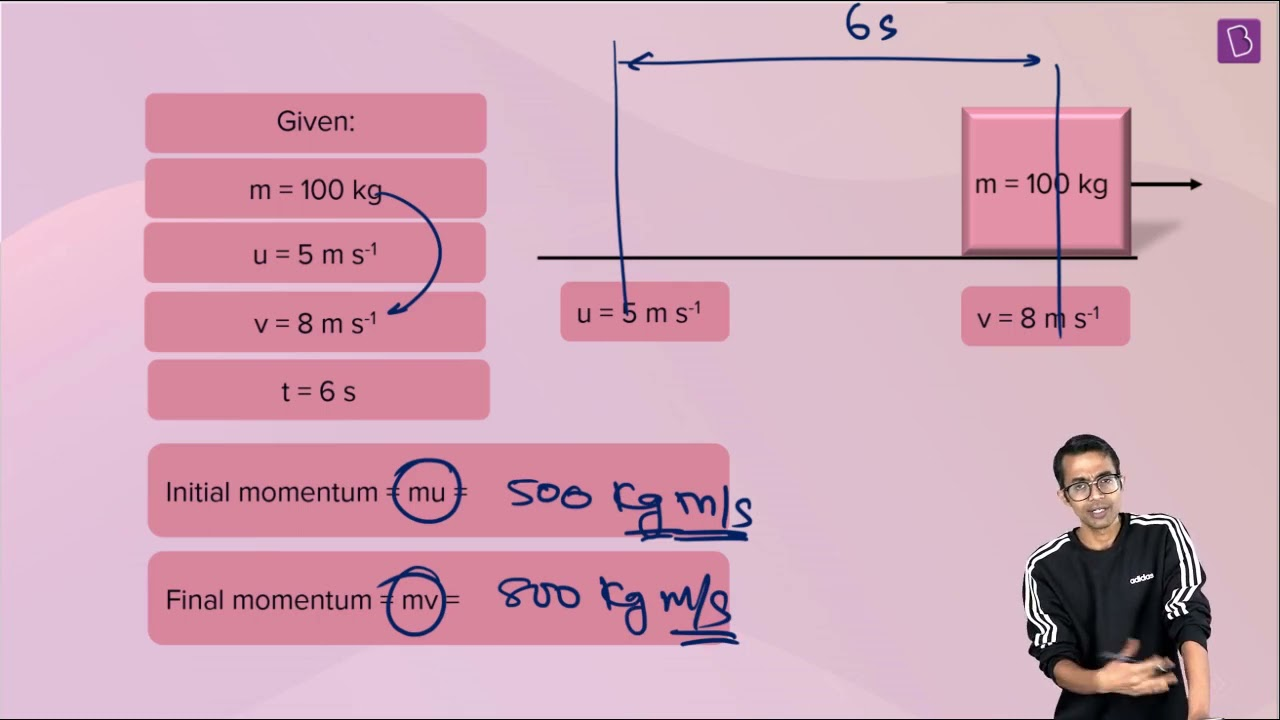17. Akhtar, Kiran, and Rahul were riding in a motorcar that was moving with a high velocity on an expressway when an insect hit the windshield and got stuck on the windscreen. Akhtar and Kiran started pondering over the situation. Kiran suggested that the insect suffered a greater change in momentum as compared to the change in momentum of the motorcar (because the change in the velocity of the insect was much more than that of the motorcar). Akhtar said that since the motorcar was moving with a larger velocity, it exerted a larger force on the insect. And as a result the insect died. Rahul while putting an entirely new explanation said that both the motorcar and the insect experienced the same force and a change in their momentum. Comment on these suggestions. Solution As per the law of conservation of momentum, the total momentum before the collision between the insect and the car is equal to the total momentum after the collision. Therefore, the change in the momentum of the insect is much greater than the change in momentum of the car (since force is proportional to mass). Akhtar’s assumption is partially right. Since the mass of the car is very high, the force exerted on the insect during the collision is also very high. Kiran's statement is false. The change in momentum of the insect and the motorcar is equal by conservation of momentum. The velocity of insect changes accordingly due to its mass as it is very small compared to the motorcar. Similarly, the velocity of motorcar is very insignificant because its mass is very large compared to the insect. Rahul’s statement is completely right. As per the third law of motion, the force exerted by the insect on the car is equal and opposite to the force exerted by the car on the insect. However, Rahul’s suggestion that the change in the momentum is the same contradicts the law of conservation of momentum. 18. How much momentum will a dumb-bell of mass 10 kg transfer to the floor if it falls from a height of 80 cm? Take its downward acceleration to be 10 ms–2. Solution Given, mass of the dumb-bell (m) = 10kg Distance covered (s) = 80cm = 0.8m Initial velocity (u) = 0 (it is dropped from a position of rest) Acceleration (a) = 10ms-2 Terminal velocity (v) =? Momentum of the dumb-bell when it hits the ground = mv As per the third law of motion v2 - u2 = 2as Therefore, v2 - 0 = 2 (10 ms-2) (0.8m) = 16 m2s-2 v = 4 m/s The momentum transferred by the dumb-bell to the floor = (10kg) × (4 m/s) = 40 kg.m.s-1 Additional Exercises Page: 130 1. The following is the distance-time table of an object in motion:
 Time (seconds) Distance (meters) 0 0 1 1 2 8 3 27 4 64 5 125 6 216 7 343
(a) What conclusion can you draw about the acceleration? Is it constant, increasing, decreasing, or zero? (b) What do you infer about the forces acting on the object? Solution (a) The distance covered by the object at any time interval is greater than any of the distances covered in previous time intervals. Therefore, the acceleration of the object is increasing. (b) As per the second law of motion, force = mass × acceleration. Since the mass of the object remains constant, the increasing acceleration implies that the force acting on the object is increasing as well 2. Two persons manage to push a motorcar of mass 1200 kg at a uniform velocity along a level road. The same motorcar can be pushed by three persons to produce an acceleration of 0.2 ms-2. With what force does each person push the motorcar? (Assume that all persons push the motorcar with the same muscular effort) Solution Given, mass of the car (m) = 1200kg When the third person starts pushing the car, the acceleration (a) is 0.2 ms-2. Therefore, the force applied by the third person (F = ma) is given by: F = 1200kg × 0.2 ms-2 = 240N The force applied by the third person on the car is 240 N. Since all 3 people push with the same muscular effort, the force applied by each person on the car is 240 N. 3. A hammer of mass 500 g, moving at 50 m s-1, strikes a nail. The nail stops the hammer in a very short time of 0.01 s. What is the force of the nail on the hammer? Solution Given, mass of the hammer (m) = 500g = 0.5kg Initial velocity of the hammer (u) = 50 m/s Terminal velocity of the hammer (v) = 0 (the hammer is stopped and reaches a position of rest). Time period (t) = 0.01s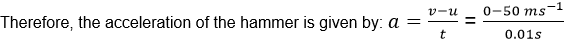a = -5000ms-2 Therefore, the force exerted by the hammer on the nail (F = ma) can be calculated as: F = (0.5kg) * (-5000 ms-2) = -2500 N As per the third law of motion, the nail exerts an equal and opposite force on the hammer. Since the force exerted on the nail by the hammer is -2500 N, the force exerted on the hammer by the nail will be +2500 N. 4. A motorcar of mass 1200 kg is moving along a straight line with a uniform velocity of 90 km/h. Its velocity is slowed down to 18 km/h in 4 s by an unbalanced external force. Calculate the acceleration and change in momentum. Also calculate the magnitude of the force required. Solution Given, mass of the car (m) = 1200kg Initial velocity (u) = 90 km/hour = 25 meters/sec Terminal velocity (v) = 18 km/hour = 5 meters/sec Time period (t) = 4 seconds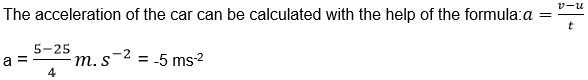Therefore, the acceleration of the car is -5 ms-2. Initial momentum of the car = m × u = (1200kg) × (25m/s) = 30,000 kg.m.s-1 Final momentum of the car = m × v = (1200kg) × (5m/s) = 6,000 kg.m.s-1 Therefore, change in momentum (final momentum – initial momentum) = (6,000 – 30,000) kg.m.s-1 = -24,000 kg.m.s-1 External force applied = mass of car × acceleration = (1200kg) × (-5 ms-2) = -6000N Therefore, the magnitude of force required to slow down the vehicle to 18 km/hour is 6000 N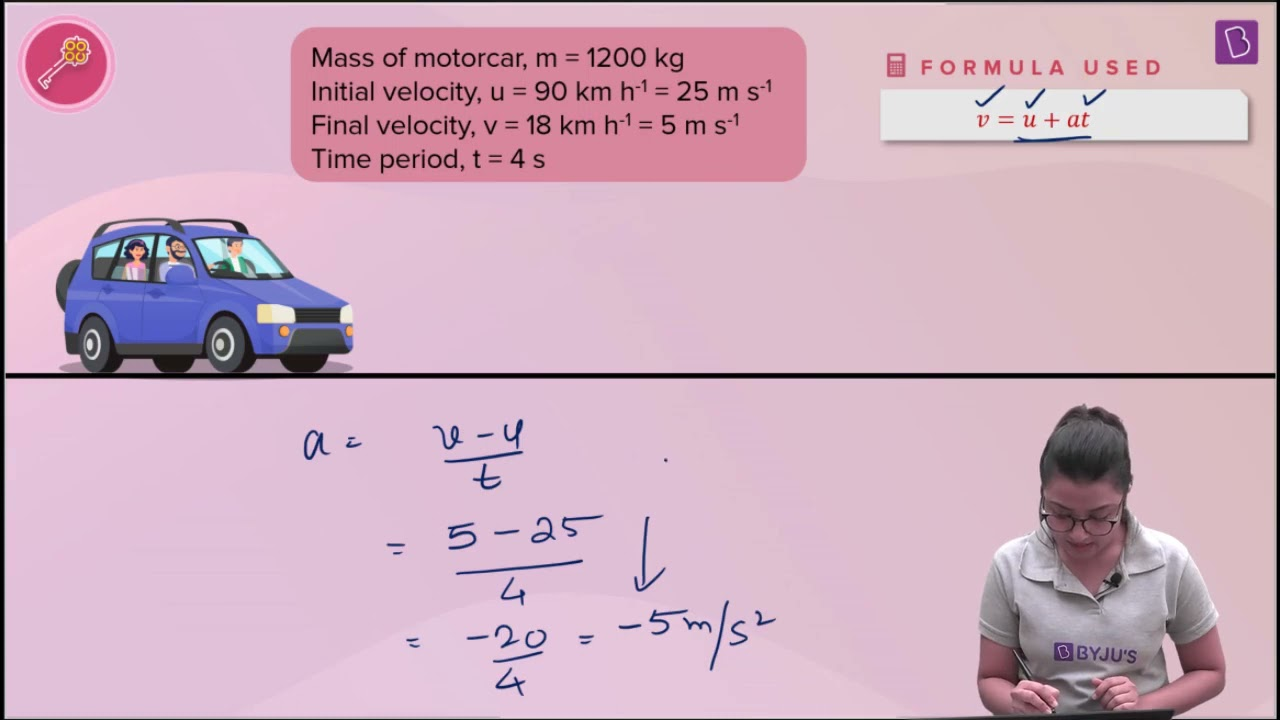## NCERT Solutions for Class 9 Science Chapter 9: Force and Laws Of Motion

NCERT Solution Class 9 Science Chapter 9 explains what is a force and its application with various examples. It also explains the three laws of motion along with examples and mathematical formulations. Conservation of Momentum is explained in a simple way. Various activities are incorporated into the chapter to explain the concepts in an easy way. NCERT Class 9 Science Chapter 9 Force and laws of motion, also describes the natural tendency of objects to resist a change in their state of rest or of uniform motion known as inertia in a detailed way. NCERT Class 9 Science Chapter 9 Force and laws of motion is covered under unit 3 Motion, Force and Work. Refer to previous years' question papers and sample papers to know the type of questions that appear along with the allotment of marks and pattern. The topics that are covered under this chapter include:

• Inertia and Mass (4 questions)
• Conservation of Momentum (4 questions)
• Post-Chapter Exercises (18 Questions)

### NCERT Solutions For Class 9 Science Chapter 9: Force and Laws Of Motion

• NCERT Solutions for Class 9 explains motion and causes of motion in a detailed way.
• 1st laws of motion, 2nd law of motion and 3rd law of motion are explained with illustrations and mathematical formulations.
• Exercise covers all the topics of the chapter and helps in gaining confidence to write the CBSE Term I exam.
• Concepts like conservation of momentum, inertia and mass are described in brief.

### Key Features of NCERT Solutions for Class 9 Science Chapter 9: Force and Laws Of Motion

• The simple and easily understandable method is followed in NCERT Solutions to make students knowledgeable of concepts.
• NCERT Solutions offer comprehensive answers to all the questions to help students in their preparations.
• Provides completely solved solutions to all the questions present in the chapter.
• These solutions will be useful for CBSE term wise exams, Science Olympiads, and other competitive exams.
[FAQ_SCHEMA_START]
Frequently Asked Questions on NCERT Solutions for Class 9 Science Chapter 9
Explain the laws of motion covered in the Chapter 9 of NCERT Solutions for Class 9 Science.
Sir Isaac Newton explained the laws of motion. There are mainly three laws of motion which is the relation between the body’s force and the motion of the body. 1. “If the body is at rest or moving at a constant speed, it will continue to rest or move at a constant speed if a force is not applied” is the first law. 2. “The acceleration of an object depends upon the force applied to the object and the mass of the object” is the second law. 3. “Every action has equal and opposite reaction” is the third law.
Which concepts in the NCERT Solutions for Class 9 Science Chapter 9 are important from the CBSE Term I exam perspective?
The concepts in the NCERT Solutions for Class 9 Science Chapter 9 which are important from the CBSE Term I exam perspective are - 1. Motion and causes of motion 2. Laws of motion with illustrations and mathematical formulations 3. Inertia and Mass 4. Conservation of Momentum.
Why should I use the NCERT Solutions for Class 9 Science Chapter 9 PDF from BYJU’S?
1. The solutions are explained in a simple and understandable way to help students with their CBSE Term I exam preparation. 2. Comprehensive answers are curated for all the questions present in the NCERT textbook. 3. NCERT Solutions are available in PDF format which can be downloaded from the links present in BYJU’S. 4. Students develop their problem solving and analytical thinking skills which are important from the exam point of view.
[FAQ_SCHEMA_END]